Courses

Statistics Previous Years Questions Notes - Class 12

Class 12: Statistics Previous Years Questions Notes - Class 12

The document Statistics Previous Years Questions Notes - Class 12 is a part of Class 12 category.
All you need of Class 12 at this link: Class 12

QUICK RECAP

• Mean:
For Discrete quantities : μ = ∑xi / N  , where N = number of quantities
For frequency distribution :  μ = ∑fixi / N
(a) If each quantity is increased or decreased by same constant then the mean gets  increased or decreased by same constant.
(b) If each quantity is multiplied or divided by same constant then the mean too gets multiplied or divided by that same constant.
• Median:
For Discrete Data : Arrange all the quantities in ascending order then,
(a) If total number of items N is odd then median = ((N + 1)/2)th term
(b) If total number of items N is even then median is the average of (N/2)th term and (N/2 + 1)th term.

For groped frequency distribution: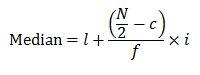where, l = exact lower limit of the median class
median class = class in which N/2 belongs
c = cumulative frequency preceding the median class
f = frequency of the median class
i = class width

• Mode:
Mode is that which is having maximum frequency. Also, if there are two classes having same maximum frequency, then Mode = 3×Median - 2×Mean (or) Median divides mode and mean in the ratio 1:2
• Mean Deviation: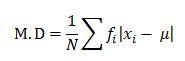• Standard Deviation(σ):
Variance = σ⇒ Standard deviation = σ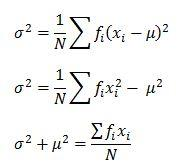(a) Standard deviation of variate (ax +b)/p is |a/p|σ
(b) If each quantity is increased or decreased by same constant "a", variance doesn't change.
(c) Coefficient of variation, CV = (σ/μ)×100
Now we will discuss some questions related to statistics which are asked in many competitive exams. At the end of there are few more self practice problems provided with key.

SOLVED PROBLEMS
Q.1. The mean of n items is μ. If the first term is increased by 1, second by 2 and so on, then what is the new mean?
Solution:
Let x1, x2,……. xn be n items.
Then, μ = [1 / n] Σ xi
Let y= x1 + 1, y2 = x2 + 2, y3 = x3 + 3,…………,yn = xn + n
Then the mean of the new series is [1 / n] Σ y= [1 / n] ∑ (x+ i)
= [1 / n] ∑ x+ 1/n (1 + 2 + 3 + ….. + n)
= μ + [1 / n] * [n (n + 1) / 2]
= μ + (n+1)/2.

Q.2. The average of n numbers x1, x2,……. xis M. If xn is replaced by x′, then what is the new average?
Solution:
M = (x1 + x2 + ... + xn)/n\frac{x_1, x_2,……. x_n}{n}
nM = x1, x2,……. xn−1 + xn
nM – x= x1, x2,……. xn−1
[nM – xn + x′] / n = [x1, x2,……. xn−1 + x′] / n
New average = [nM − xn + x′] / n

Q.3. Mean of 100 observations is 45. It was later found that two observations 19 and 31 were incorrectly recorded as 91 and 13. The correct mean is _________.
Solution:
Sum of 100 items = 45 × 100 = 4500
Sum of items added = 19 + 31 = 50
Sum of items replaced = 91 + 13 = 104
New sum = 4500 − 104 + 50 = 4446
New mean= 4446 / 100 = 44.46

Q.4. The following data gives the distribution of the height of students.

 Height (in cm) 160 150 152 152 161 154 155 Name of students 12 8 4 4 3 3 7

What is the median of the distribution?
Solution:
Arranging the data in ascending order of magnitude, we obtain

 Height (in cm) 150 152 154 155 156 160 161 Number of students 8 4 3 7 3 12 4 Cumulative frequency 8 12 15 22 25 37 41

Here, the total number of items is 41 i.e., an odd number.
Hence, the median is [(41 + 1) / 2]th i.e., 21st item.
From the cumulative frequency table, we find that median i.e., 21st item is 155.
(All items from 16 to 22nd are equal, each 155).

Q.5. The mean and S.D. of the marks of 200 candidates were found to be 40 and 15 respectively. Later, it was discovered that a score of 40 was wrongly read as 50. The correct mean and S.D. respectively are ______________.
Solution:
Corrected Σx = 40 × 200 − 50 + 40 = 7990
Corrected x = 7990 / 200 = 39.95
Correct Σx= 365000 − 2500 + 1600 = 364100
Corrected σ = √((364100/200) − (39.95)2)
= √(1820.5 − 1596)
= √224.5
= 14.98

Q.6. If a variable takes the discrete values α − 4, α − 7 / 2, α − 5 / 2, α − 3, α − 2, α + 1 / 2, α − 1 / 2, α + 5 (α > 0), then the median is ____________.
Solution:
Arrange the data as α − 7/2, α − 3, α − 5/2, α − 2, α − 1/2, α + 1/2, α – 4, α + 5
Median = [1 / 2] [value of 4th item + value of 5th item ]
Median = [(α − 2) + (α − 1 / 2)] / 2
= [2α − 5 / 2] / 2
= α − 5 / 4

Q.7. The median of a set of 9 distinct observations is 20.5. If each of the largest 4 observations of the set is increased by 2, then the median of the new set is _______.
Solution:
Since n = 9, then median term = ([9 + 1] / 2)th = 5th term.
Now, the last four observations have increased by 2.
The median is 5th observation, which is remaining unchanged.
There will be no change in the median.

Q.8. Runs scored by a batsman in 10 innings are: 38, 70, 48, 34, 42, 55, 63, 46, 54, 44 the mean deviation about median is ___________.
Solution:
Arranging the given data in ascending order, we have 34, 38, 42, 44, 46, 48, 54, 55,63, 70, Median, M = [46 + 48] /  = 47
(Here, n = 10, median is the mean of 5th and 6th items)
Mean deviation = (∑xi - M)/N = (13 + 9 + 5 + 3 + 1 + 1 + 7 + 8 + 16 + 23)/10
= 8.6

Q.9. Computer the median from the following table.

 Marks obtained No. of students 0-10 2 10-20 18 20-30 30 30-40 45 40-50 35 50-60 20 60-70 6 70-80 3

Solution:

 Marks obtained No. of students Cumulative frequency 0-10 2 2 10-20 18 20 20-30 30 50 30-40 45 95 40-50 35 130 50-60 20 150 60-70 6 156 70-80 3 159

N = Σf = 159 (Odd number)
Median is [1 / 2] (n + 1) = [1 / 2] [(159 + 1)] = 80th value, which lies in the class [30 – 40] (see the row of cumulative frequency 95, which contains 80).
Hence the median class is [30 – 40].
We have l = Lower limit of median class = 30
f = frequency of median class = 45
C = Total of all frequencies preceding median class = 50
i = width of class interval of median class=10
Required median = l + ([N / 2 − C] / f) * i
= 30 + ([159 / 2 − 50] / 45) × 10
= 3 + 295 / 45
= 36.55

Q.10. The number of observations in a group is 40. If the average of the first 10 is 4.5 and that of the remaining 30 is 3.5, then the average of the whole group is _________.
Solution:
[x1 + x2 + ….. + x10] /  = 4.5
[x1 + x2 + ….. + x10] = 45 and [x11 + x12 +….. +x40] /  = 3.5
x11 + x12 +….. + x40 = 105
x1 + x2 + ….. + x40 = 150
[x1 + x2 + ….. + x40] /  = 150 / 40 = 15 / 4.

Q.11. A school has four sections of chemistry in class XII having 40, 35, 45 and 42 students. The mean marks obtained in chemistry tests are 50, 60, 55 and 45 respectively for the four sections, the overall average of marks per student is _____________.
Solution:

Total number of students = 40 + 35 + 45 + 42 = 162
Total marks obtained = (40 × 50) + (35 × 60) + (45 × 55) + (42 × 45) = 8465
Overall average of marks per student = 8465 / 162 = 52.25.

Q.12. The mean weight per student in a group of seven students is 55 kg If the individual weights of 6 students are 52, 58, 55, 53, 56 and 54; then weights of the seventh student is __________.
Solution:
Total weight of 7 students is = 55× 7 = 385 kg
Sum of weight of 6 students = 52 + 58 + 55 + 53 + 56 + 54 = 328 kg
Weight of seventh student = 385 – 328 = 57kg.

Q.13. In a class of 100 students there are 70 boys whose average marks in a subject are 75. If the average marks of the complete class are 72, then what are the average marks of the girls?
Solution:
Let the average marks of the girls students be x, then
72 = [70 × 75 + 30 x] / 100
or [7200 − 5250] /  = x
x = 65.

Q.14. The average weight of students in a class of 35 students is 40 kg. If the weight of the teacher is included, the average rises by 12kg; the weight of the teacher is __________.
Solution:
Let the weight of the teacher is w kg , then
40 + [1 / 2] = [35 × 40 + w] / [35 + 1]
36 × 40 + 36 × 12 = 35 × 40 + w
w=58
Weight of the teacher = 58kg.

Q.15. In an experiment with 15 observations on x, the following results were available ∑x2 = 2830 ∑x = 170.On observation that was 20 was found to be wrong and was replaced by the correct value 30. Then the corrected variance is
(a) 78.00
(b) 188.66
(c) 177.33
(d) 8.33
Solution: (a)
New ∑x = a = 170 - 20 + 30 = 180
New ∑x2 = b = 2380 - 400 + 900 = 3330
σ2 + a2 = b2/15
σ2 + 1802 = 33302/15
σ2 = 78

Q.16. The means of five observations is 4 and their variance is 5.2. If three of these observations are 1, 2 and 6, then the other two are
(a) 2 and 9
(b) 3 and 8
(c) 4 and 7
(d) 5 and 6
Solution: (c)
Let the two unknown numbers be x,y
Mean = a = 4 = (1 + 2 + 6 + x + y)/5
x + y = 11 --- (1)
Variance = b = 5.2
c = ∑x2 = 1 + 4 + 36 + x2 + y2 = 41 + x2 + y2
b + a2 = c/5
5(5.2 + 16) = 41 + x2 + y2
x2 + y2 = 65 --- (2)
On solving (1) and (2) we get x = 4 and y = 7

Q.17. If the mean deviation of the numbers 1, 1 +d, 1 + 2d, ... , 1 + 100d from their mean is 225, then a value of "d" is
(a) 10.1
(b) 20.2
(c) 10
(d) 5.05
Solution: (a)
Let μ be the mean and MD be the mean deviation from the mean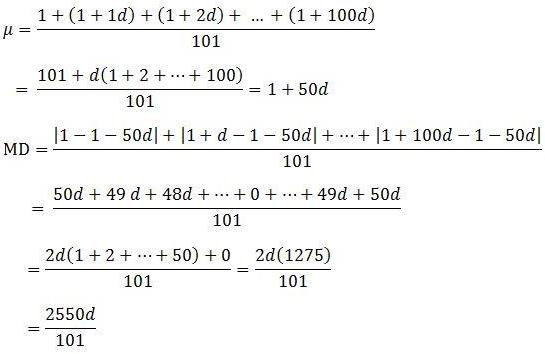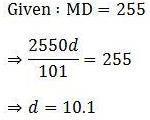Q.18. If the standard deviation of the numbers 2, 3, a and 11 is 3.5 then which of the following is true?
(a) 3a2 - 26a + 55 = 0
(b) 3a2 - 32a + 84 = 0
(c) 3a2 - 34a + 91 = 0
(d) 3a2 - 23a + 44 = 0
Solution: (b)
σ = 3.5 = 7/2 ⇒ σ2 = 49/4
μ = (2 + 3 + a + 11)/4 = (16 + a)/4
y = ∑xi2 = 4 + 9 + a2 + 121 = 134 + a2
σ2 + μ2 = y/5
On keeping the above values and solving we get,
3a2 - 32a + 84 = 0

Q.19. The mean age of 25 teachers in a school is 4 years. A teacher retires at the age of 60 years an a new teacher is appointed in his place. If the new mean age of the teachers in the school is 39 then the age (in years) of the newly appointed teacher is _______.
Solution: 35
Let the new teacher's age be "x"
Initial mean = 40
Initial total of ages = 25 x 40 = 1000
Final total of ages = 1000 - 60 + x = 940 + x
New mean = (940 + x)/25 = 39
⇒ 940 + x = 25 x 39
⇒ x = 25

Q.20. If ∑(xi - 5) = 9 and ∑(xi - 5)= 45 where i = 1,2,3, ... , 9 then the standard deviation of these 9 quantities is _______.
Solution: 2
Note that there are a total of 9 quantities
∑(xi - 5) = 9
∑xi - 5 x 9 = 9 ⇒ ∑xi = 54
μ = 54/9 = 6
∑(xi2 - 10xi + 25) = 45
∑xi2 - 10∑xi + 25 x 9 = 45
∑xi2 - 10(54) + 225 = 45 ⇒ ∑xi2 = 360
Variance = σ2
σ2 + μ2 = ∑xi2/9
σ2 + 62 = 360/9
σ2 = 40 - 36 = 4
Standard deviation = σ = 2

Q.21. The mean of a set of 30 observations is 75. If each other observation is multiplied by a non-zero number λ and then each of them is decreased by 25, their mean remains same. The value of λ equals to _______.
Solution: 4/3
Initial mean = 75
The mean successively changes as follows,
75 → 75λ → (75λ - 25)
Given, initial mean = final mean
75 = 75λ - 25
⇒ λ = 4/3

Q.22. If the sum of the deviations of 50 observations from 30 is 50 then the mean of these observations is ______.
Solution: 31
Number of observations = 50
Sum of deviations = ∑(x1 - 30) = 50
(x1 - 30) + (x2 - 30) + ... + (x50 - 30) = 50
∑xi - 30(50) = 50
∑xi = 1550
μ = ∑xi/50 = 1550/50 = 31

Q.23. The mean and variance of 7 observations are 8 and 16 respectively. If 5 of the observations 2,4,10,12,14 then the product of the remaining two observations is ______.
Solution: 48
Number of observations = 7
Let the remaining two numbers be a,b
μ = 8 ⇒ ∑xi = 8 x 7 = 56
2 + 4 + 10 + 12 + 14 + a + b = 56 ⇒ a + b = 14
σ= 16
σ2 + μ2 = ∑xi2/7
16 + 64 = ∑xi2/7 ⇒ ∑xi2 = 560
4 + 16 + 100 + 144 + 196 + a2 + b2 = 560
a2 + b2 = 100
(a + b)2 - 2ab = 100
2ab = 196 - 100 = 96
⇒ ab = 48

Q.24. If the standard deviation of the numbers -1,0,1,k is √5 where k > 0. The the value of k is equal to ______.
Solution: 2√6
σ = √5 ⇒ σ2 = 5
Mean, μ = (-1 + 0 + 1 + k)/4 = k/4
y = ∑xi2 = 1 + 0 + 1 + k2 = k2 + 2
σ2 + μ2 = y/4
5 + k2/16 = (k2 + 2)/4
k = 2√6

Q.25. The mean and the median of the following 10 numbers (in increasing order) 10,22,26,29,34,x,42,67,70,y are 42 and 35. Then the value y/x is equal to ______.
Solution: 7/3
Number of terms = 10
So, median = average of 5th and 6th terms
35 = (34 + x)/2 ⇒ x = 36
Mean = (10 + 22 + 26 + 29 + 34 + 36 + 42 + 67 + 70 + y)/10 = 42
336 + y = 420 ⇒ y = 84
y/x = 84/36 = 7/3

Q.26. If for some x∈R, the frequency distribution of the marks obtained by 20 students in a test is

 Marks 2 3 5 7 Frequency (x + 1)2 2x - 5 x2 - 3x x

The the mean of marks  is ______.
Solution: 2.8
Number of students = ∑fi = 20
(x + 1)2 + 2x - 5 + x2 - 3x + x = 20
x2 + x - 12 = 0
x = 3, -4
Since on substituting x = -4 some of the frequencies are becoming negative x = -4 is excluded. So x = 3

 Marks 2 3 5 7 Frequency 16 1 0 3

Mean = (2 x 16 + 3 x 1 + 5 x 0 + 7 x 3)/20
= 2.8

Q.27. There are 10 numbers such that the mean of first four numbers is 11 and the mean of the remaining 6 is 16. If the sum of the squares of all these is 2000, then the standard deviation of this data is _____.
Solution: 2
Total of first four observations = 4 x 11 = 44
Total of next 6 observations = 6 x 16 = 96
Mean = s = (44 + 96)/10 = 14
y = ∑xi2 = 2000
σ2 + s2 = y/10
σ2 + 196 = 200
σ = 2

PRACTICE PROBLEMS
Q.1. The mean of 5 observations is 5 and their variance is 124. If three of the observations are 1,2 and 6 find the mean deviation from the mean of the data.

Q.2. The sum of 100 observations and the sum of their squares are 400 and 2475 respectively. Later on three observations 3,4 and 5 were found incorrect. If the incorrect observations are omitted, then find the variance of the remaining observation.

Q.3. 5 students of a class have an average height 150 cm and variance 18 cm2. A new student, whose height is 156 cm joined them. Find the the new variance (in cm2) of these six students.

Q.4. In a group of data, there are n observations. If ∑(xi + 1)2 = 9n and ∑(xi - 1)2 = 5n find the standard deviation of this data.

Q.5. The mean of 5 observations is 5 and their variance is 9.2 . If three of the given data are 1,3 and 8 then find the ratio of the other two observations.

Q.6. A student scores 45,54,41,57,43 in 5 tests. His score is not known for the sixth test but his average score for all 6 tests is 48. Find the standard deviation of his marks in all the six tests.
KEY
(1) 2.8
(2) 9
(3) 20
(4) √5
(5) 4:9
(6) 10/√3

The document Statistics Previous Years Questions Notes - Class 12 is a part of Class 12 category.
All you need of Class 12 at this link: Class 12Use Code STAYHOME200 and get INR 200 additional OFF Use Coupon Code

Track your progress, build streaks, highlight & save important lessons and more!

,

,

,

,

,

,

,

,

,

,

,

,

,

,

,

,

,

,

,

,

,

;